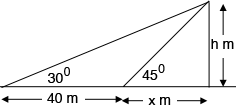SEARCH HOMEMath Central Quandaries & QueriesQuestion from nombulelo, a student: the shadow of a tower,when the angle of elevation of the sun is 30degrees is found to be 40 meters longer than when it is 45 degrees. Find the height of the towerHi Nombulelo,

In my diagram the height of the tower is $h$ meters.What trig function relates the height $h$ metres, the side length $x$ metres and the angle measurement $45^o?$

What trig function relates the height $h$ metres, the side length $40 + x$ metres and the angle measurement $30^o?$

This gives you two equations in $h$ and $x.$ Solve for $h.$

PennyMath Central is supported by the University of Regina and The Pacific Institute for the Mathematical Sciences.